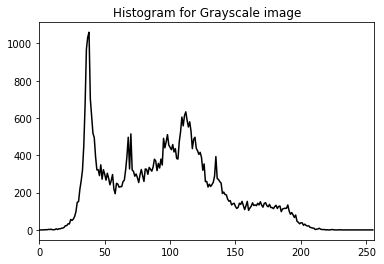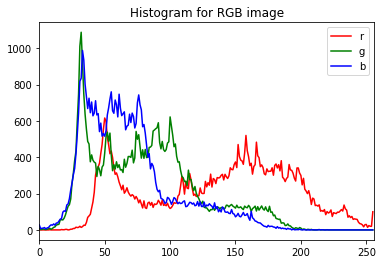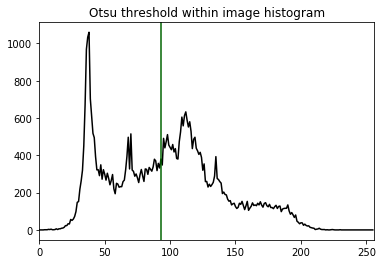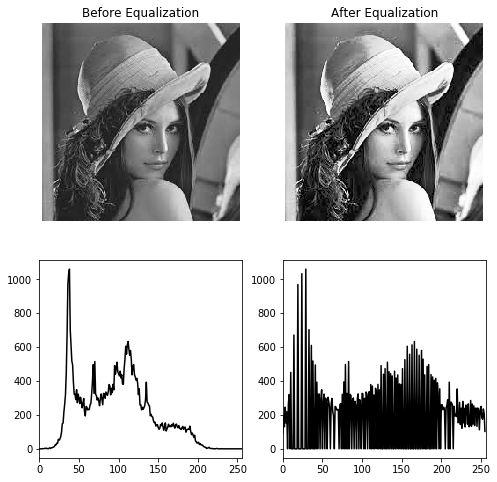Notebook

# Digital Image Processing

In :
%run load_lib_ch2.py


## Chapter 2: Histograms

A histogram is a graphical representation of the distribution of tones in a digital image.

The number of pixels of each intensity value is plotted in bins.

The total number of pixels in each bin can be normalized by dividing by the total number of pixels in the image to determine the probabiliy of occurance of each intensity.

These histograms are often used for thresholding by analyzing the peaks and valleys and extracting the optimal thresholds.

In :
plt.imshow(img)
plt.axis('off')
plt.show()In :
grayscale_hist(img)In :
rgb_hist(img)It can be observed that the grayscale histogram is roughly equal to $(Red+2.Green+Blue)/4$ and therefore has the most resemblance to the Green channel histogram.

### Otsu's method

Otsu's method is a widely used algorithm in Computer Vision and Image Processing. It is named after Professor Nobuyuki Otsu. It automatically computes the optimal threshold to binarize a grayscale image. It assumes that the image contains two classes of pixel.

In :
otsu_img = otsu(img)In :
otsu_hist(img)### Histogram Equalization

Histogram equalization is a technique for adjusting pixel intensities to enhance the contrast of the image. As seen in the example below, the histogram is more spread apart, while the first image had no pixels with high intensities, the second one does.

In :
hist_equ_example(img)Equalization works the following way:

Algorithm for Histogram Equalization 1) Calculate probability of each intensity 2) Calculate cumulative probability 3) Multiply cumulative probability by maximum intensity
• 1) The probability $p_n$ of each pixel intensity is calculated as the number of pixels with that intensity $n$ divided by the total number of pixels.

• 2) The cummulative probability is calculated by summing all previous pixel probabilities. For example for pixel intensity 10, the cummulative probability is the sum of all pixel probabilities for intensities 0-10. $c_n = \sum_{i=0}^{i=n}p_i$

• 3) The cummulative probabilities are multiplied by the maximum pixel intensity (255 in this case). The result is the new intensity of all pixels.